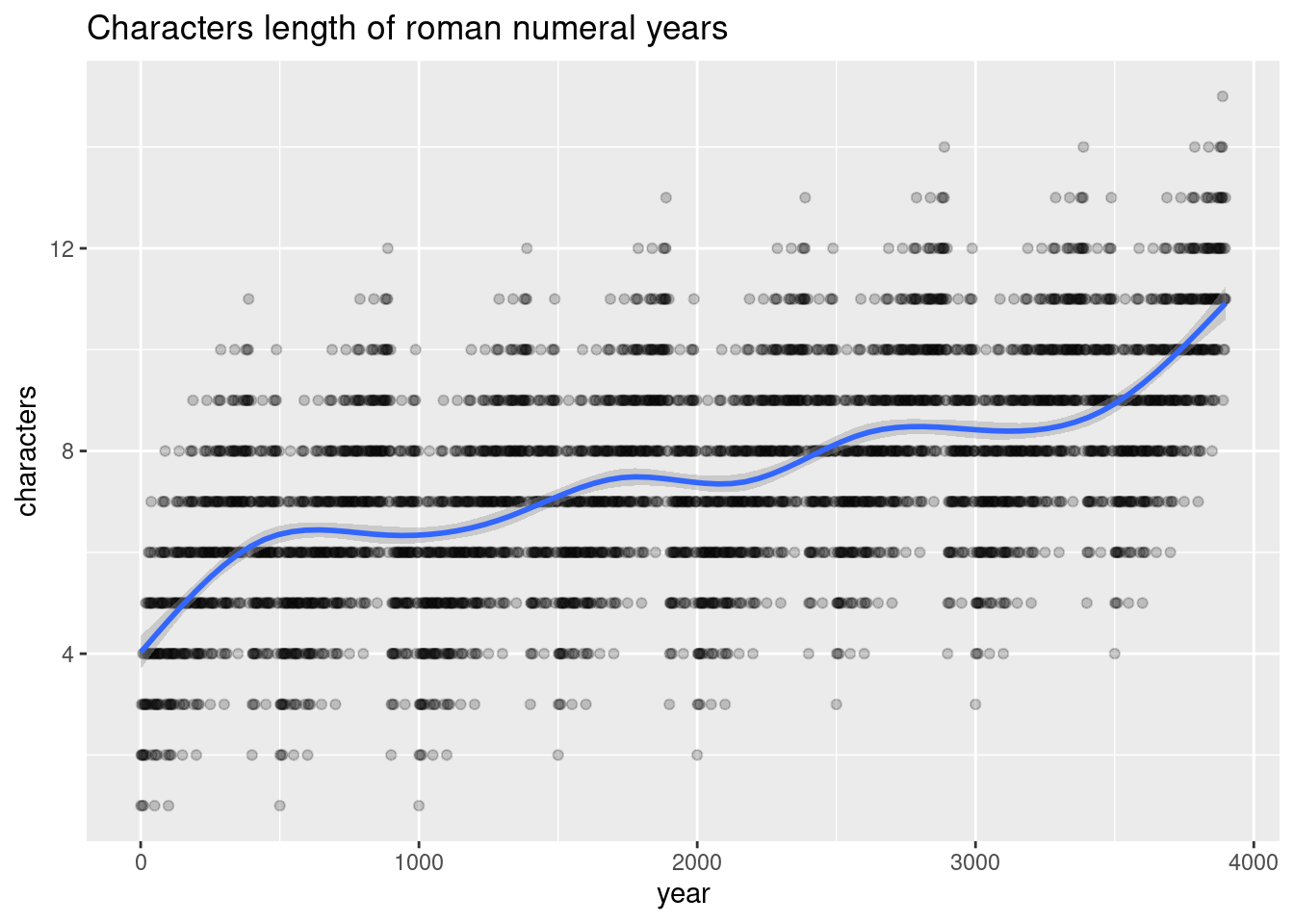# Playing with Roman numerals

R
Author

Michaël

Published

2020-12-25

Modified

2023-10-03

What is the longest year number (yet) written in Roman numerals?

``````library(tidyverse)

tibble(y = 1:2020) |>
mutate(r = as.roman(y),
l = str_length(r)) |>
slice_max(l)``````
``````# A tibble: 1 × 3
y r                 l
<int> <roman>       <int>
1  1888 MDCCCLXXXVIII    13``````

It is year 1888, with 13 characters…

And the largest writable number being 3899, according to the strict rules in R (however some say it’s 3999),

``````tibble(y = 1:5000) |>
mutate(r = as.roman(y),
l = str_length(r)) |>
slice_min(l)``````
``````# A tibble: 1 × 3
y r               l
<int> <roman>     <int>
1  3899 MMMDCCCXCIX    11``````

the longest overall year will be year 3888 with 15 characters.

``````tibble(y = 1:3899) |>
mutate(r = as.roman(y),
l = str_length(r)) |>
slice_max(l)``````
``````# A tibble: 1 × 3
y r                   l
<int> <roman>         <int>
1  3888 MMMDCCCLXXXVIII    15``````

Nice pattern:

``````tibble(y = 1:3899) |>
mutate(r = as.roman(y),
l = str_length(r)) |>
ggplot(aes(y, l)) +
geom_point(alpha = .2) +
geom_smooth() +
labs(title = "Characters length of roman numeral years",
x = "year",
y = "characters")``````And there are only eleven palindromic years:

``````tibble(y = 1:3899) |>
mutate(r = as.character(as.roman(y)),
rr = stringi::stri_reverse(r)) |>
filter(r == rr,
str_length(r) > 1)``````
``````# A tibble: 11 × 3
y r     rr
<int> <chr> <chr>
1     2 II    II
2     3 III   III
3    19 XIX   XIX
4    20 XX    XX
5    30 XXX   XXX
6   190 CXC   CXC
7   200 CC    CC
8   300 CCC   CCC
9  1900 MCM   MCM
10  2000 MM    MM
11  3000 MMM   MMM  ``````